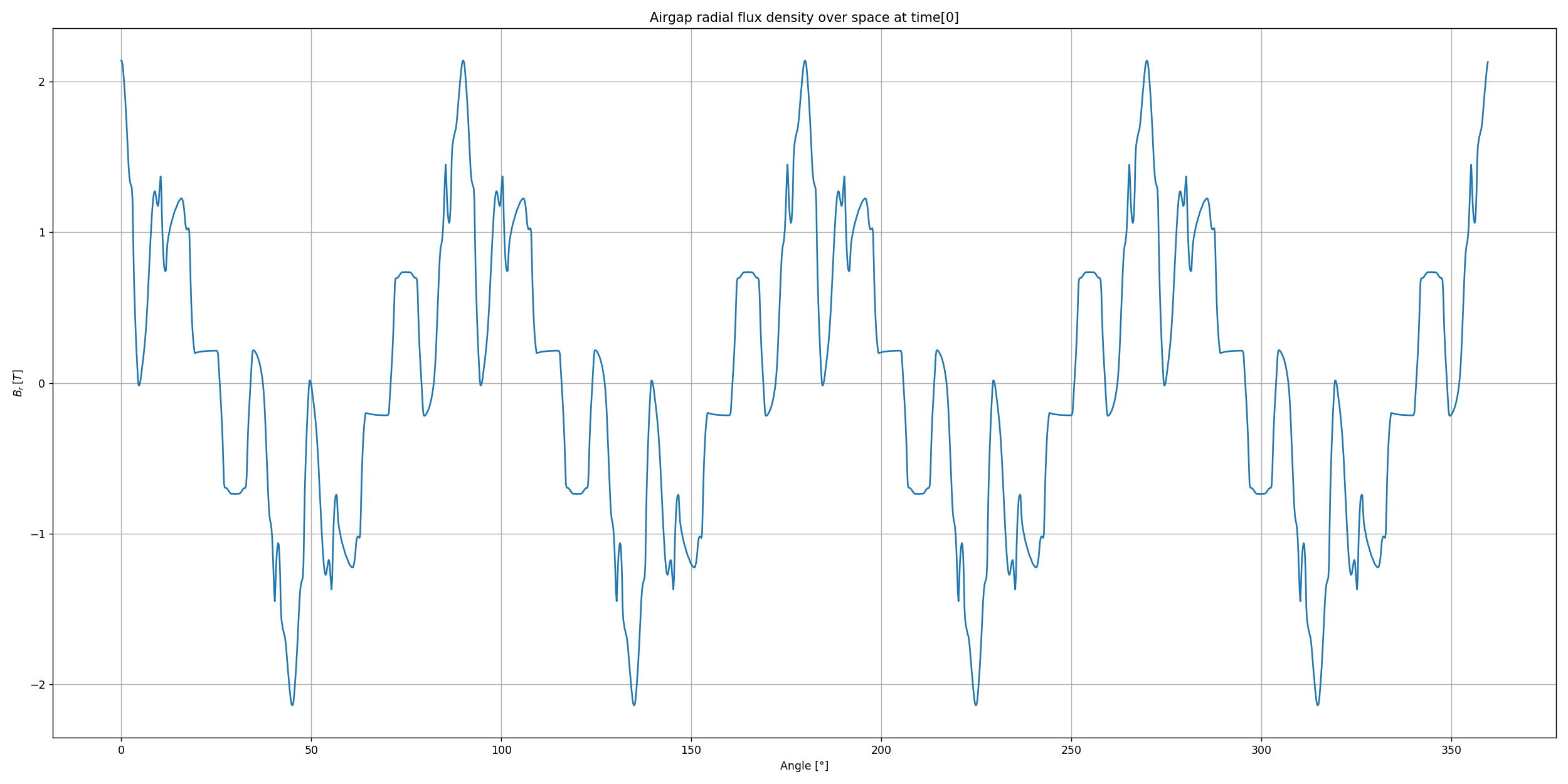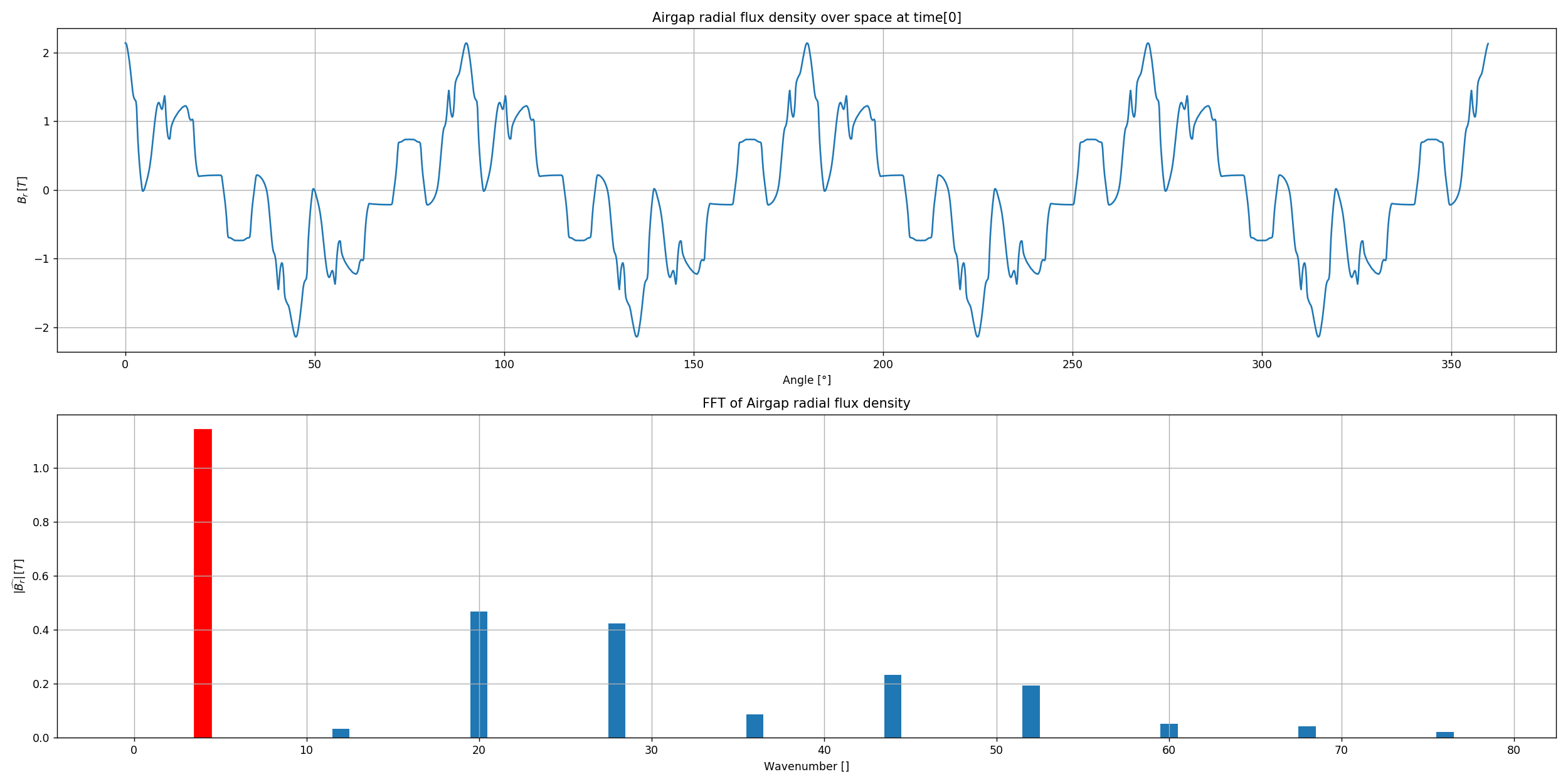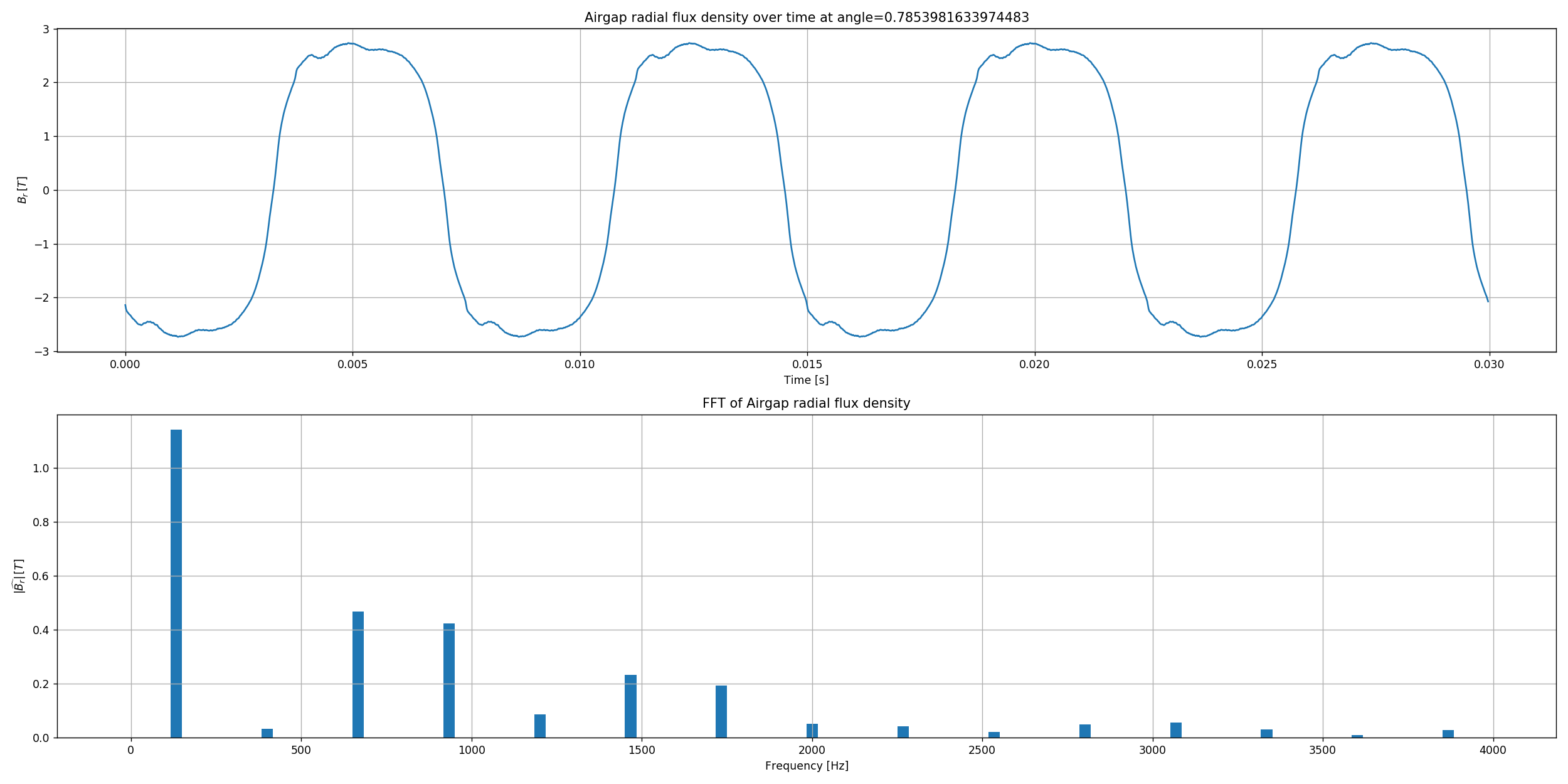# How to plot results¶

Pyleecan has generic built-in plot methods which allow to easily plot:

• simulated or experimental fields (unit conversions supported)
• slices (normalizations available)
• Fourier Transforms

but also to compare on the same graph a list of fields.

This flexibility is provided by the SciDataTool module, which has been created to ease the handling of scientific data, and considerately simplify plot commands. It unifies the extraction of relevant data (e.g. slices), whether they are stored in the time/space or in the frequency domain. The call to Fourier Transform functions is transparent, although it still can be parameterized.

This tutorial explains how to use Pyleecan’s plot commands. It is available on GitHub. For more details on the SciDataTool package, please refer to the specific tutorials:

The first step consists in getting simulation data. In order to demonstrate the capabilities of the plot commands, a simulation over 2048 time steps has been performed in the simulation tutorial, and the resulting Output object has been stored on Pyleecan’s website. First, these results need to be downloaded:

:

# Change of directory to have pyleecan in the path
from os import chdir
chdir('..')

# Import Pyleecan modules

import urllib.request
url = 'https://www.pyleecan.org/Data/tuto_Simu_FEMM_results.json'
urllib.request.urlretrieve(url, 'tuto_Simu_FEMM_results.json')
print("Done")

# Load Output object from json file
print("Done")

Downloading the simulation data...
Done
Done


The following example demonstrates the syntax to quickly plot the airgap radial flux density obtained at the end of the simulation:

:

%matplotlib notebook
#------------------------------------------------------
# Plot the flux density as a function of space
out.plot_A_space("mag.Br")
#------------------------------------------------------The plot command requires the name of the field (“mag.Br” for instance). Titles and legends are automatically generated. In this tutorial, only the airgap radial flux density is plotted, but the commands work with any field computed in Pyleecan.

There are, for the time being, 7 plot commands in Pyleecan:

• plot_A_space: field as a function of the angle
• plot_A_time: field as a function of time
• plot_A_surf: 3D surface in time/space domain
• plot_A_time_space: 3D surface + angle + time
• plot_A_nthoct: spectrum in the $$1/n^{\text{th}}$$ octave band
• plot_A_cfft2: 3D stem plot of the 2D Fourier Transform
• plot_A_fft2: pcolor plot of the 2D Fourier Transform

## plot_A_space¶

### Description¶

This plot command plots a field as a function of the angle, at a given time step (first time step by default, or value prescribed by the user). It can also add a subplot with the space Fourier Transform if the is_fft key is activated.

### Parameters¶

Parameter Description Type Default Value
Data_str Name of the field str mandatory
t Slice at time=t float None
t_index Slice at time[t_index] int 0
is_deg Angle in degrees or radians bool True
is_fft Add the fft as a subplot bool False
is_spaceorder x-axis of the fft in space orders bool False
r_max Maximum value for the wavenumbers to display int 100
fund_harm Frequency of the fundamental harmonic float None
is_norm Normalize the field bool False
unit Unit of the field str "SI"
out_list List of Output objects to compare [Output] []

### Example¶

:

#------------------------------------------------------
# Plot the flux density as a function of space with the spatial fft
r_max = 78
out.plot_A_space("mag.Br", is_fft=True, r_max=r_max)
#------------------------------------------------------## plot_A_time¶

### Description¶

This plot command plots a field as a function of time, at a given angle (first angle by default, or value prescribed by the user). It can also add a subplot with the Fourier Transform if the is_fft key is activated.

### Parameters¶

Parameter Description Type Default Value
Data_str Name of the field str mandatory
alpha Slice at angle=alpha float None
alpha_index Slice at angle[alpha_index] int 0
is_fft Add the fft as a subplot bool False
is_elecorder x-axis of the fft in electrical orders bool False
freq_max Maximum value for the frequencies to display float 20000
is_norm Normalize the field bool False
unit Unit of the field str "SI"
out_list List of Output objects to compare [Output] []

### Example¶

:

from numpy import pi

#------------------------------------------------------
# Plot the flux density as a function of time with the time fft
freq_max = 4000
out.plot_A_time("mag.Br", alpha=pi/4, is_fft=True, freq_max=freq_max)
#------------------------------------------------------## plot_A_surf¶

### Description¶

This plot command plots a field as a 3D surface in the time/space domain.

### Parameters¶

Parameter Description Type Default Value
Data_str Name of the field str mandatory
is_deg Angle in degrees or radians bool True
t_max Maximum value for time float 1.0
a_max Maximum value for space float 400
z_max Maximum value for the field float 1.0
is_norm Normalize the field bool False
unit Unit of the field str "SI"
colormap Colormap cmp "RdBu_r"

### Example¶

:

#------------------------------------------------------
# Plot the flux density as a surface over time and space
t_max = 0.03
z_max = 3.0
out.plot_A_surf("mag.Br", t_max=t_max, z_max=z_max)
#------------------------------------------------------